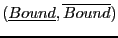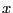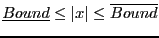Next: Example Up: First Cauchy theorem Previous: Mathematical background   Contents

Implementation

This procedure is implemented as:

int Cauchy_First_Bound_Interval(int Degree,VECTOR &Coeff,INTERVAL &Bound);
with:
• Degree: degree of the polynomial
• Coeff: the Degree+1 coefficients of the polynomial in increasing degree
• Bound: the interval on the absolute value of the roots
This procedure returns 0 if Degree=0, Coeff(1)=0 or Coeff(Degree+1)=0 and Degree=1. On success the return code is 1. There is also an implementation for interval polynomial:

int Cauchy_First_Bound_Interval(int Degree,INTERVAL_VECTOR &Coeff,INTERVAL &Bound);
This procedure returns an intervalsuch that for any polynomial in the set and for any rootof this polynomial. A failure code of 0 is returned if Coeff(1) or Coeff(Degree+1) contains 0 or if Degree=0. On success the returned code is 1.

Jean-Pierre Merlet 2012-12-20